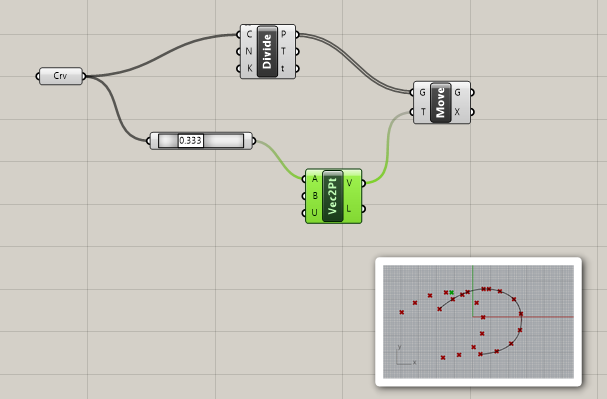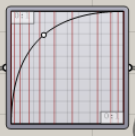# Divide curve and march the points along the curve

Hi, extreme newbie here.

I’d like to get points along a curve, then march the points along the curve.

I’ve had a naïve go, but this moves all the points as a set, so they are all simultaneously undergoing the same linear translation (as I guess is obvious).

Can I somehow split out the points, so they can all independently follow the original curve?

TIAWhere should go the last point? Outside the curve?

Your “Point on curve” component, set at 0.333 is evaluating a point along your curve (probably at something like 13,1,0) , and then you created a vector from that point to an unset B input, (0,0,0), resulting in a -13,1,0 vector.
You just moved all your points, then…

Some approach would be to use the tangents for each point (T), giving amplitude and moving the points.
Or shattering the curve with the t parameters and then evaluating each segment differently.

Why are you wanting to do so? It’s something… unusual.

Why wouldn’t you just divide the curve where you need the points? Rather than dividing the curve then moving the points like you want to do.

Did you try Divide length component?

Thanks people.

I’m trying to animate a rope being pulled along, passing over a pulley.

The ‘points source’ curve would be a helix representing the lay of the rope fibres.

I couldn’t think of a way of distorting the helix as it passed over the pulley, so hit on the idea of breaking it down into hundreds points, each one to follow the ‘driving curve’. The driving curve being a straight section, then semi circular round the pulley and another straight section.

This a learning exercise, but still hopefully with a useful outcome.

I’m probably trying to run before I can walk…

This sounds promising. I’ll look into the difference between Shatter and Divide.

And doing it with two curves connecting the points…

Awsome! Thanks Ftzuk.

Could you help me out with the name of this component:Cheers, Ian

Graph mapper

1 Like

Thanks Filipe.